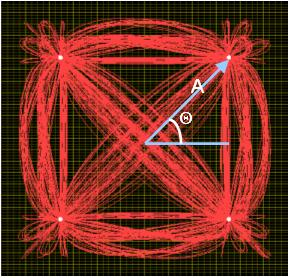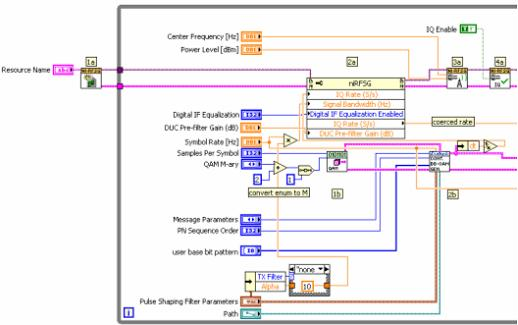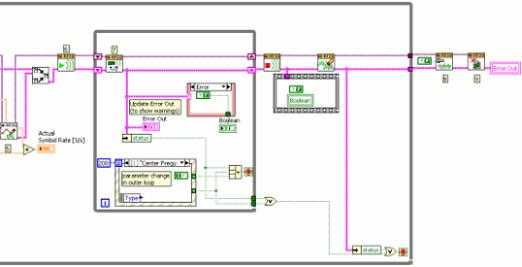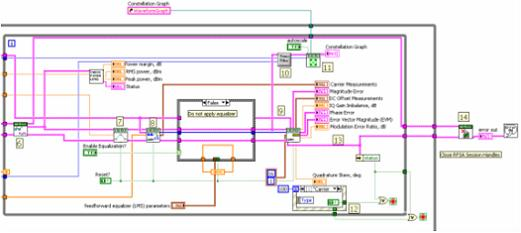# RF Hardware Example: QAM

Publish Date: Aug 05, 2009 | 1 Ratings | 5.00 out of 5 | Print | Submit your review

## Overview

This lab is designed to examine the Quadrature Amplitude Modulation (QAM) digital modulation scheme. Fundamentally, digital modulation requires changing characteristics of the carrier wave over time. Each change results in a sine wave with a different phase, amplitude, or frequency than before. As a result, different “states” of the sine wave are referred to as symbols which represent some digital bit pattern. In this exercise, we will construct LabVIEW VIs that transmit and receive a digital bit stream using QAM.

### Background:

Each symbol in the QAM symbol map or constellation plot is distinguished by a unique phase and amplitude.  In addition, the M-ary of the modulation scheme defines how many symbols are in the specific symbol map.  For example, 4-QAM has a symbol map with 4 symbols and 256-QAM has a symbol map of 256 symbols.  A constellation plot illustrating 4-QAM is shown below.  With QAM modulation, the IQ data is mapped to actual symbols.  Note that each of the symbols are represented by the four white dots on the graph.  As the annotations on the graph illustrate, each symbol can be represented by polar coordinates consisting of an amplitude (A) and a phase (Θ).When QAM modulation is used by the transmitter, both the phase and amplitude of the carrier wave are changed over time to represent the transmission of digital data.   Thus, with 4-QAM modulation, there are exactly four combinations of phase and amplitude of the carrier wave.  Each combination of phase and amplitude represents a unique symbol.  In addition, each symbol represents a unique set of binary information.  With 4-QAM modulation, each symbol represents two bits of information.  When sending an actual bit stream, every two bits are grouped to generate the corresponding symbol.  For example, the bit stream (0, 1, 0, 0, 1, 0) would be grouped into three symbols: (01, 00, 10).  Thus, three symbols are required to represent the entire bit stream.  This is in contrast to 64-QAM which contains 64 unique symbols.  As a result of the high symbol number, each symbol in 64 QAM can be represented by 6 bits.  Thus, the bit stream (0, 1, 0, 0, 1, 0) can be represented by one symbol (010010).  The affect of this phenomenon is that as M-ary increases, more bits can be represented by each symbol.

### Programming:

Generation

1a. Open a session to the NI-RFSG.

1b. Calculate parameters for use with the MT Modulate QAM VI.

2a. Query the waveform parameters.

2b. Generate phase continuous QAM signal with complete PN cycle.

3. Configure the frequency and output power.

4. Enable IQ Generation.5. Resample the QAM signal and write it.

6. Initiate generation according to programmed settings.

7. Check the generation status and exit if an error has occurred.

8. Disable the output. This sets the noise floor as low as possible.

9. Close the session to the NI-RFSG.Acquisition

1. Initialize the RF downconverter and the digitizer.

2. Set the QAM system parameters.

3. Set the reference level of the 5600 to the expected max input level, for best signal to noise. Configure the horizontal subsystem of the digitizer.  The MT Configure Downconversion Settings VI takes in the user parameters and determines the correct sample rate and number of samples to acquire.

4. Generate the filter coefficients for the demodulator.5. Determine a bandwidth based on the input symbol rate and filter parameters.  The 1.1 factor in the default case provides a small cushion for carrier offset.

6. Acquire and downconvert (if necessary).  The data coming out of the 5620 can be IF or interleaved I - Q, depending on whether the DDC is used.  The data coming out of MT Convert IF to IQ is always I - Q represented as a complex signal.

7. Resample the signal.  The demodulator requires an integer number of samples per symbol.  MT Fractional Resample resamples the data to satisfy this condition.  Set the new sample rate to Fs' = (symbol rate)*(samples per symbol).

8. Demodulate.  The "recovered waveform" output of the demodulator is equivalent to the input with frequency and phase offsets removed, and the first sample corresponds to an ideal symbol time.

9. Calculate measurements on the recovered waveform.

10. Truncate the filter transient.  This is only necessary if the filter is Root Raised Cosine, but the code is simpler if we always truncate.

11. Format and display the constellation plot.

12. Use the Event Structure to control the loop structures.  A time-out event (Event ) reads the "stop" button, and all loops will exit if the "stop" = TRUE. If stop is FALSE, and there is no error, the inner loop will run again.  If any controls in the middle loop change, Event  will fire,  the inner loop will exit, and the middle loop will run again.  If any controls in the outer loop change, Event  will fire, both the inner and middle loops will exit, and the outer loop will run again.

13. Measurement errors do not stop inner loop. We want to be able to look at an arbitrary signal without stopping the application.

14. If the middle loop exits, the instrument handles and the receiver info will be closed.  The new handles will be opened if the outer loop runs again.### Bookmark & Share

Attachments:

RFSA QAM Eye Diagram

Requirements

RFSA QAM Demodulate

Requirements

RFSG Generate QAM

Requirements

### Ratings

Rate this document